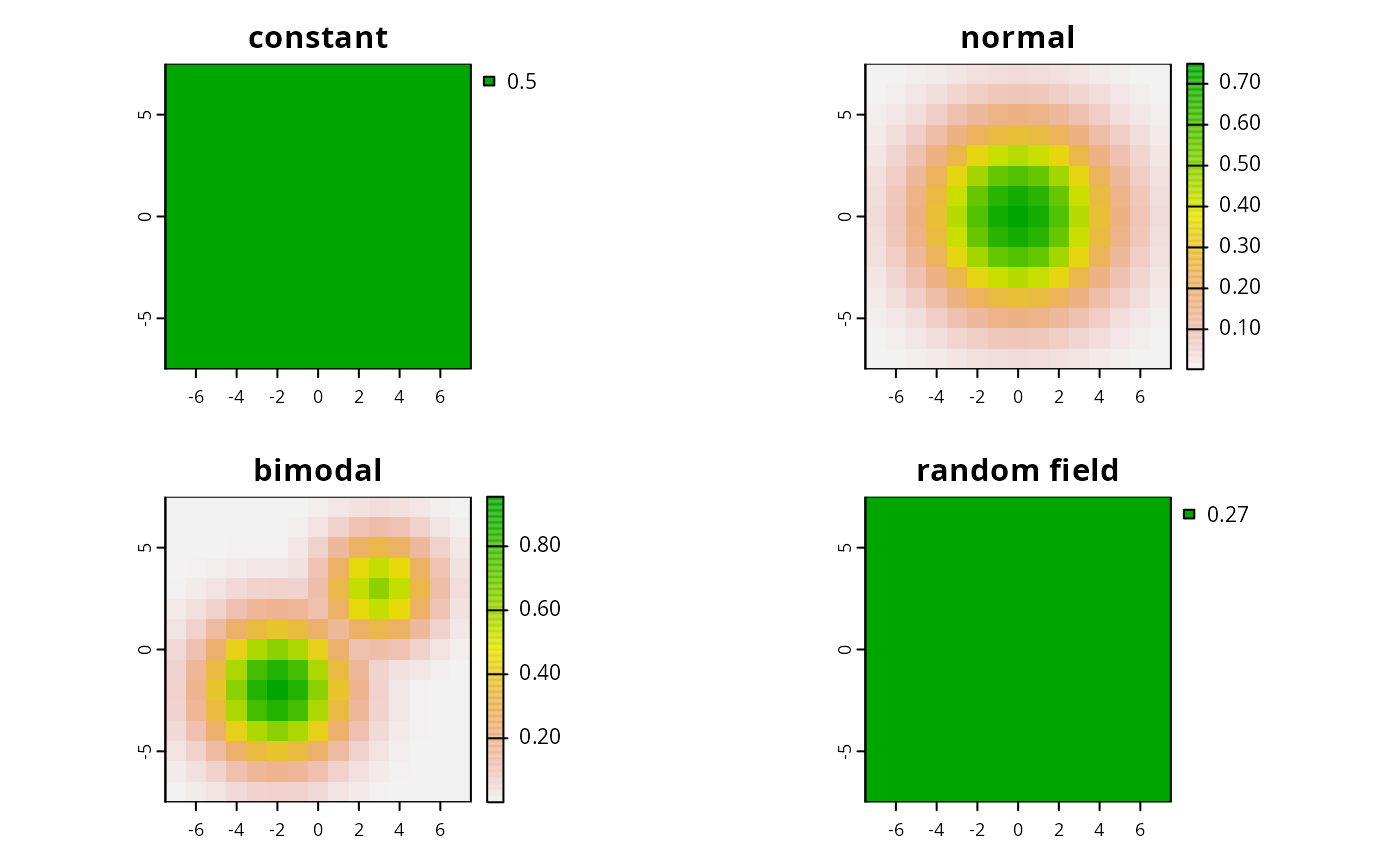This function simulates species distributions for RAP.

sim.species(x, ...)

# S3 method for RasterLayer
sim.species(
x,
n = 1,
model = list("uniform", "normal", "bimodal", RandomFields::RPgauss())[],
...
)

# S3 method for SpatialPolygons
sim.species(
x,
res,
n = 1,
model = list("normal", "uniform", "bimodal", RandomFields::RPgauss())[],
...
)

## Arguments

x raster::raster() or sp::SpatialPolygons() object delineate the spatial extent to delineate study area. parameters passed to RandomFields::RandomFields(). integer number of species. Defaults to 1. RandomFields::RMmodel() model to simulate species distributions with. Defaults RandomFields::RPgauss(). numeric resolution to simulate distributions. Only needed when sp::SpatialPolygons() supplied.

## Value

raster::stack() with layers for each species.

## Details

Distributions are simulated by passing model to RandomFields::RFsimulate() and converting to logistic values using boot::inv.logit().

RandomFields::RFsimulate().

## Examples

# make polygons
sim_pus <- sim.pus(225L)

# simulate 1 uniform species distribution using RasterLayer
s1 <- sim.species(blank.raster(sim_pus, 1), n = 1, model = "uniform")

# simulate 1 uniform species distribution based on SpatialPolygons
s2 <- sim.species(sim_pus, res = 1, n = 1, model = "uniform")

# simulate 1 normal species distributions
s3 <- sim.species(sim_pus, res = 1, n = 1, model = "normal")

# simulate 1 bimodal species distribution
s4 <- sim.species(sim_pus, res = 1, n = 1, model = "bimodal")

# simulate 1 species distribution using a RModel object from RandomFields
s5 <- sim.species(sim_pus, res = 1, n = 1, model = RandomFields::RPgauss())

# simulate 5 species distribution using a RModel object from RandomFields
s6 <- sim.species(sim_pus, res = 1, n = 5, model = RandomFields::RPgauss())#> .....
# plot simulations
par(mfrow = c(2,2))
plot(s2, main = "constant")
plot(s3, main = "normal")
plot(s4, main = "bimodal")
plot(s5, main = "RPgauss()")